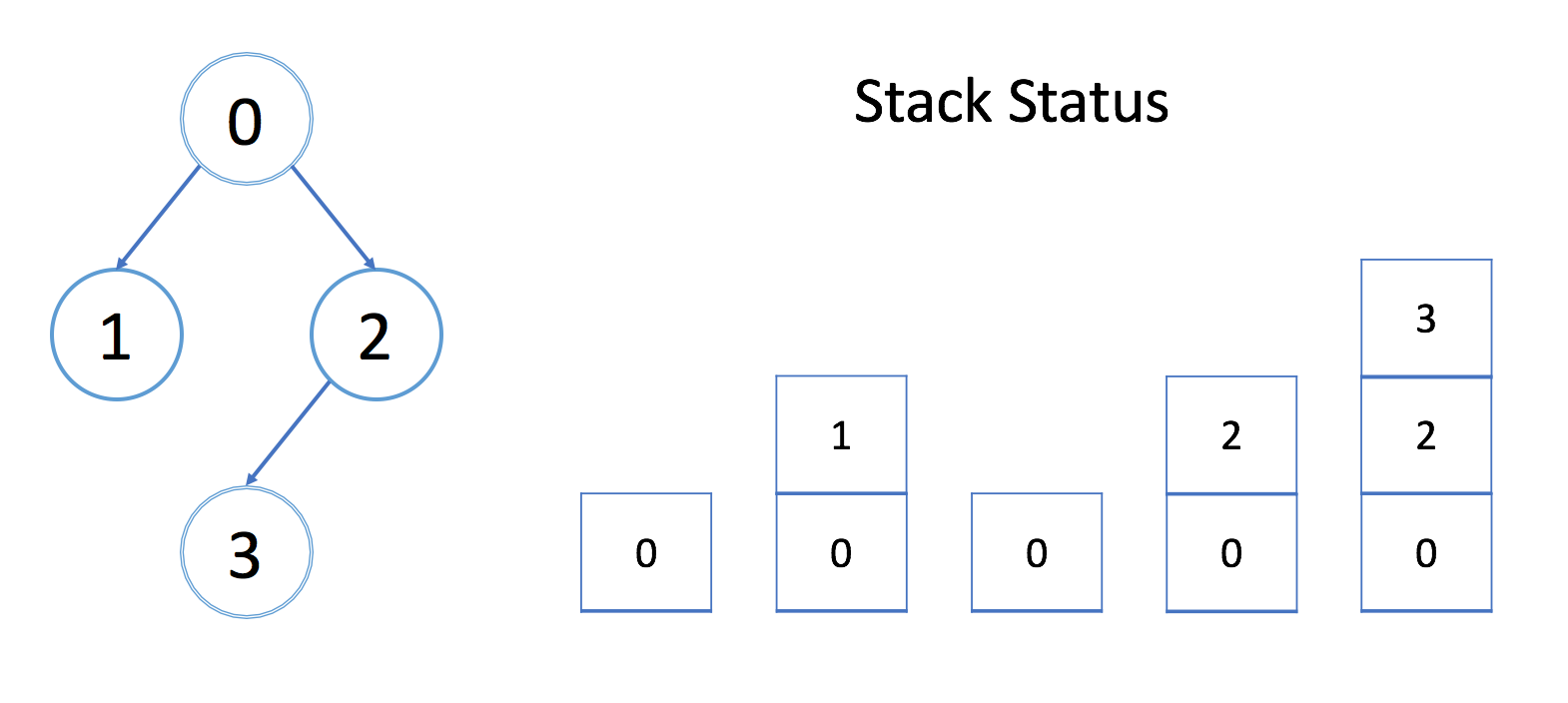网站从因情语写改为晴雨            这个网站的模板也从calmlog_ex改为 whimurmur

## leetcode探索之队列 & 栈学习 栈和深度优先搜索

549人浏览 / 0人评论 / | 作者：因情语写  | 分类： 设计模式与算法  | 标签： 设计模式与算法  /  leetcode  |

先决条件：树的遍历

与 BFS 类似，深度优先搜索（DFS）是用于在树/图中遍历/搜索的另一种重要算法。也可以在更抽象的场景中使用。

正如树的遍历中所提到的，我们可以用 DFS 进行 前序遍历中序遍历后序遍历。在这三个遍历顺序中有一个共同的特性：除非我们到达最深的结点，否则我们永远不会回溯。

这也是 DFS 和 BFS 之间最大的区别，BFS永远不会深入探索，除非它已经在当前层级访问了所有结点。

通常，我们使用递归实现 DFS。递归中起着重要的作用。在本章中，我们将解释在执行递归时栈的作用。我们还将向你展示递归的缺点，并提供另一个没有递归的 DFS 实现。

在准备面试时，DFS 是一个重要的话题。DFS 的实际设计因题而异。本章重点介绍栈是如何在 DFS 中应用的，并帮助你更好地理解 DFS 的原理。要精通 DFS 算法，还需要大量的练习。

## 栈和 DFS

与 BFS 类似，深度优先搜索（DFS）也可用于查找从根结点到目标结点的路径。在本文中，我们提供了示例来解释 DFS 是如何工作的以及栈是如何逐步帮助 DFS 工作的。

### 示例

我们来看一个例子吧。我们希望通过 DFS 找出从根结点 A 到目标结点 G 的路径。### 洞悉

观看上面的动画后，让我们回答以下问题：

1. 结点的处理顺序是什么？

在上面的例子中，我们从根结点 A 开始。首先，我们选择结点 B 的路径，并进行回溯，直到我们到达结点 E，我们无法更进一步深入。然后我们回溯到 A 并选择第二条路径到结点 C 。从 C 开始，我们尝试第一条路径到 E 但是 E 已被访问过。所以我们回到 C 并尝试从另一条路径到 F。最后，我们找到了 G。

总的来说，在我们到达最深的结点之后，我们只会回溯并尝试另一条路径。

2. 栈的入栈和退栈顺序是什么？

如上面的动画所示，我们首先将根结点推入到栈中；然后我们尝试第一个邻居 B 并将结点 B 推入到栈中；等等等等。当我们到达最深的结点 E 时，我们需要回溯。当我们回溯时，我们将从栈中弹出最深的结点，这实际上是推入到栈中的最后一个结点。

结点的处理顺序是完全相反的顺序，就像它们被添加到栈中一样，它是后进先出（LIFO）。这就是我们在 DFS 中使用栈的原因。

## DFS - 模板 I

正如我们在本章的描述中提到的，在大多数情况下，我们在能使用 BFS 时也可以使用 DFS。但是有一个重要的区别：遍历顺序。

与 BFS 不同，更早访问的结点可能不是更靠近根结点的结点。因此，你在 DFS 中找到的第一条路径可能不是最短路径。

在本文中，我们将为你提供一个 DFS 的递归模板，并向你展示栈是如何帮助这个过程的。在这篇文章之后，我们会提供一些练习给大家练习。

### 模板 - 递归

有两种实现 DFS 的方法。第一种方法是进行递归，这一点你可能已经很熟悉了。这里我们提供了一个模板作为参考：

``````/*
* Return true if there is a path from cur to target.
*/
boolean DFS(Node cur, Node target, Set<Node> visited) {
return true if cur is target;
for (next : each neighbor of cur) {
if (next is not in visited) {
return true if DFS(next, target, visited) == true;
}
}
return false;
}``````

当我们递归地实现 DFS 时，似乎不需要使用任何栈。但实际上，我们使用的是由系统提供的隐式栈，也称为调用栈（Call Stack）。

### 示例

让我们看一个例子。我们希望在下图中找到结点 0 和结点 3 之间的路径。我们还会在每次调用期间显示栈的状态。在每个堆栈元素中，都有一个整数 cur，一个整数 target，一个对访问过的数组的引用和一个对数组边界的引用，这些正是我们在 DFS 函数中的参数。我们只在上面的栈中显示 cur。

每个元素都需要固定的空间。栈的大小正好是 DFS 的深度。因此，在最坏的情况下，维护系统栈需要 O(h)，其中 h 是 DFS 的最大深度。在计算空间复杂度时，永远不要忘记考虑系统栈。

## 算法-岛屿数量

给定一个由 '1'（陆地）和 '0'（水）组成的的二维网格，计算岛屿的数量。一个岛被水包围，并且它是通过水平方向或垂直方向上相邻的陆地连接而成的。你可以假设网格的四个边均被水包围。

``````输入:
11110
11010
11000
00000

``````输入:
11000
11000
00100
00011

``````class Solution {
// 想法，每出现陆地，结果加1，将相邻陆地置'0'，还有另一种解法：https://blog.csdn.net/weixin_40550726/article/details/82943658
public int numIslands(char[][] grid) {
int result = 0;
for(int i = 0; i < grid.length; i++){
for(int j = 0; j < grid.length; j++){
if(grid[i][j] == '1'){
result++;
}
}
}

return result;
}

private void maskIsland(int i, int j, char[][] grid){
if(i < 0 || i >= grid.length || j < 0 || j >= grid.length || grid[i][j] == '0'){
return;
}

grid[i][j] = '0';

}
}``````

算法思想在注释中，每遇到一块陆地，陆地数加1，该陆地及相邻陆地变海洋，那么再遇到陆地时一定是不相邻的陆地，对该陆地进行相同的操作。

## 算法-克隆图

``````class Node {
public int val;
public List<Node> neighbors;
}``````

``````输入：adjList = [[2,4],[1,3],[2,4],[1,3]]

``````输入：adjList = [[]]

``````输入：adjList = []

``````输入：adjList = [,]

1. 节点数介于 1 到 100 之间。
2. 每个节点值都是唯一的。
3. 无向图是一个简单图，这意味着图中没有重复的边，也没有自环。
4. 由于图是无向的，如果节点 p 是节点 q 的邻居，那么节点 q 也必须是节点 p 的邻居。
5. 图是连通图，你可以从给定节点访问到所有节点。
``````/*
// Definition for a Node.
class Node {
public int val;
public List<Node> neighbors;

public Node() {}

public Node(int _val,List<Node> _neighbors) {
val = _val;
neighbors = _neighbors;
}
};
*/
class Solution {
// 想法：维护一个visited列表，先处理当前结点的所有相邻结点，然后相同作法处理所有相邻结点, 将原节点与新节点进行
// 映射，每访问一个原结点中的未访问节点，同步新建一个节点，对于节点的邻居，如果已有映射，用映射的节点，否则建新节点并进行
// 新老映射
public Node cloneGraph(Node node) {
Set<Node> visited = new HashSet<>();
Map<Node, Node> map = new HashMap<>();
Deque<Node> queue = new ArrayDeque<>();
List<Node> nbs;
Node temp, newTemp, nb, newNode = null;
boolean first = true;

while(!queue.isEmpty()){
temp = queue.removeFirst();
if(!visited.contains(temp)){
if(map.get(temp) == null){
newTemp = new Node(temp.val, null);
map.put(temp, newTemp);
}else{
newTemp = map.get(temp);
}

if(first){
newNode = newTemp;
first = false;
}

if(null != temp.neighbors){
nbs = new ArrayList<>();
for(Node n: temp.neighbors){
if(map.get(n) == null){
nb = new Node(n.val, null);
map.put(n, nb);
}else{
nb = map.get(n);
}

if(!visited.contains(n)){
}
}

newTemp.neighbors = nbs;
}

}

}

return newNode;
}

}``````

上面用的是广度优先算法，用到了Map, Set, List, Deque，比较复杂，下面再看一种比较快的方法。

``````/*
// Definition for a Node.
class Node {
public int val;
public List<Node> neighbors;

public Node(int _val) {
val = _val;
neighbors = new ArrayList<Node>();
}
}
*/
class Solution {
public Node cloneGraph(Node node) {
Map<Node, Node> lookup = new HashMap<>();
return dfs(node, lookup);
}

private Node dfs(Node node, Map<Node,Node> lookup) {
if (node == null) return null;
if (lookup.containsKey(node)) return lookup.get(node);
Node clone = new Node(node.val, new ArrayList<>());
lookup.put(node, clone);
return clone;
}

}``````

上面用到了深度优先算法，深搜的好处是可以自动处理所有结点，而不用像广搜那样显示加入队列中处理

## 算法-目标和

``````输入: nums: [1, 1, 1, 1, 1], S: 3

-1+1+1+1+1 = 3
+1-1+1+1+1 = 3
+1+1-1+1+1 = 3
+1+1+1-1+1 = 3
+1+1+1+1-1 = 3

1. 数组非空，且长度不会超过20。
2. 初始的数组的和不会超过1000。
3. 保证返回的最终结果能被32位整数存下。
``````class Solution {
// 想法：总共可能有2的length次方个，可以用一个二进制数表示，每一位0表示负数，1表示正数：超时
/*public int findTargetSumWays(int[] nums, int S) {
int bin, len = nums.length, sum, temp,mark,result = 0;
bin = 1<<len;

for(int i = bin - 1; i >= 0; i--){
sum = 0;
for(int j = 0; j < len; j++){
temp = 1<<j;
mark = i & temp;
if(mark == 0){
sum -= nums[j];
}else{
sum += nums[j];
}
}

if(sum == S){
result++;
}
}

return result;
}*/

// 想法：每个子部分和原来的结构相同，想到可以用递归，动态规划
public int findTargetSumWays(int[] nums, int S) {
return findTargetSumWays(nums, S, 0, nums.length - 1);
}

private int findTargetSumWays(int[] nums, int S, int i, int j){
if(j - i == 0){
if(nums[i] == S && nums[i] == -S){
return 2;
}else if(nums[i] == S || nums[i] == -S){
return 1;
}

return 0;
}

return findTargetSumWays(nums, S - nums[i], i + 1, j) + findTargetSumWays(nums, S + nums[i], i + 1, j);
}
}``````

利用了部分结果与整体结果的关系，总的方法数等于各种情况下方法数的和，递归分治

## DFS - 模板 II

递归解决方案的优点是它更容易实现。 但是，存在一个很大的缺点：如果递归的深度太高，你将遭受堆栈溢出。 在这种情况下，您可能会希望使用 BFS，或使用显式栈实现 DFS。

这里我们提供了一个使用显式栈的模板：

``````/*
* Return true if there is a path from cur to target.
*/
boolean DFS(int root, int target) {
Set<Node> visited;
Stack<Node> s;
while (s is not empty) {
Node cur = the top element in s;
return true if cur is target;
for (Node next : the neighbors of cur) {
if (next is not in visited) {
}
}
remove cur from s;
}
return false;
}``````

该逻辑与递归解决方案完全相同。 但我们使用 while 循环和栈来模拟递归期间的系统调用栈。 手动运行几个示例肯定会帮助你更好地理解它。

## 算法-二叉树的中序遍历

``````输入: [1,null,2,3]
1
\
2
/
3

``````/**
* Definition for a binary tree node.
* public class TreeNode {
*     int val;
*     TreeNode left;
*     TreeNode right;
*     TreeNode(int x) { val = x; }
* }
*/
class Solution {
// 递归
/* public List<Integer> inorderTraversal(TreeNode root) {
List<Integer> result = new ArrayList<>();

if(null != root){
inorderTraversal(root, result);
}

return result;
}

public void inorderTraversal(TreeNode node, List<Integer> result){
if(node.left != null){
inorderTraversal(node.left, result);
}

if(node.right != null){
inorderTraversal(node.right, result);
}
}*/

// 用栈，非递归
public List<Integer> inorderTraversal(TreeNode root) {
Deque<TreeNode> stack = new ArrayDeque<>();
List<Integer> result = new ArrayList<>();
Set<TreeNode> visited = new HashSet<>();
TreeNode temp, left;

if(root != null){
stack.push(root);

while(!stack.isEmpty()){
temp = stack.peek();
while(temp.left != null && !visited.contains(temp.left)){
stack.push(temp.left);
temp = temp.left;
}

stack.pop();

if(null != temp.right){
stack.push(temp.right);
}
}
}

return result;
}
}``````

上面是用递归和用栈两种情况下进行中序遍历